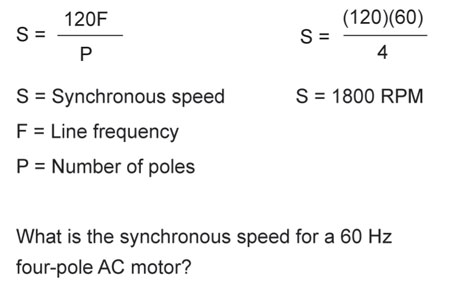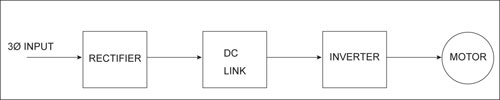# Speed Control of Motors — An Introduction to Variable-Frequency Drives

Speed, torque, and horsepower are three interrelated parameters in motor control. The speed of a motor measured in revolutions per minute (RPM) defines a motor’s ability to spin at a rate per unit time. The torque of a motor measured in foot-pounds (ft-lb) is a rotational characteristic of the motor that is the algebraic product of force multiplied by distance. Electrically, one horsepower (hp) is equal to 746 watts. What is interesting about these motor parameters is that if you change one of the three variables, the other two are affected. For example, if you increase horsepower while keeping speed constant, torque increases.

An electric motor is a device that converts electrical energy into mechanical energy. An electrical signal is applied to the input of the motor and the output of the motor produces a defined amount of torque related to the characteristics of the motor. It is important to understand speed-torque characteristic curves as they show the relationship between speed as a percent of rated speed versus load torque as a percent of full rating. Motors are available in multi-speed configurations that can provide constant torque variable horsepower, constant horsepower variable torque, and variable torque variable horsepower.Equation 1. Synchronous speed

Traditionally, dc motors have been used in precise speed control applications because of their ability to provide acceleration and deceleration from a dead stop position to full speed fairly easily. In a dc series motor (the field is in series with the armature), the speed is controlled by increasing or decreasing the applied voltage to the series field or armature by means of a field rheostat or an armature rheostat. Silicon controlled rectifiers (SCRs) have replaced rheostats as they can control large blocks of power without the heat dissipation problems of carbon or wire-wound variable resistors. Additionally, SCRs are much smaller in size than their earlier counterparts and interface well with programmable logic controllers.

The AC squirrel cage induction motor is essentially a constant speed device. The speed of the rotating magnetic field is referred to as synchronous speed. Equation 1 relates the synchronous speed (S) to the incoming line frequency (F) and number of poles (P) the machine is constructed of: [S = 120(F) ÷ P].

Here’s an example to illustrate this point. In the United States, the line frequency is 60 Hertz. A four-pole AC squirrel cage induction motor would therefore have a synchronous speed of 1800 RPM [(120 x 60) ÷ 4]. In practice, the motor will run at less than 1800 RPM as load is placed on the rotor. This difference in speed between synchronous speed and full-load speed is referred to as slip and is usually expressed as a percentage. Note that the only two variables in equation 1 that define speed are the incoming line frequency and the number of poles in the machine. Since the number of poles in a machine is fixed, the only variable that is left to change is the incoming line frequency. This is the basis for the operation of a variable-frequency drive (VFD).

It is important to understand the difference between the AC and the DC machine at this point. Earlier, we mentioned that a DC machine could have its speed changed by increasing or decreasing the applied voltage. This is not the case for an AC motor. In fact, you can damage an AC squirrel cage induction motor if you vary the incoming supply voltage.

The term variable-frequency drive is often used interchangeably with AC drive, inverter, or adjustable- frequency drive (AFD). The two most common circuits for adjusting the speed of an AC squirrel cage induction motor are the inverter and the cycloconverter.

The VFD using an inverter does two things: first, it takes the incoming AC signal and converts it to a DC signal through a process known as rectification, and then it takes the rectified DC signal and inverts it back to a variable voltage and variable-frequency AC signal. An inverter takes a waveform like a rectified DC signal and generates an equivalent time-varying waveform resembling a sinusoid. A block diagram for an inverter type VFD is shown in figure 1.Figure 1. Block diagram of PWM VFD

The VFD using a cycloconverter is a device that produces an AC signal of constant or controllable frequency from a variable-frequency AC signal input. The output frequency is usually one-third or less than the input frequency. The cycloconverter type of VFD is normally used with larger motors or groups of motors at once.

Typical specifications you might encounter with an inverter-type VFD are listed below:

Horsepower: 1–10 HP @ 230V

Input frequency: 50/60 Hz

Output frequency: 0–120 Hz standard; 0–400 Hz jumper selectable

Frequency setting potentiometer: 10 K ½ W

Ambient temperature: 0 – +40° C

Control method: PWM (pulse width modulation)

Transistor type: IGBT (insulated gate BJT)

Analog outputs: assignable

Digital Outputs: opto-isolated assignable

Terminal strips present on the VFD allow the device to interface to the outside world to familiar switching devices like start, stop, forward run, and reverse run. Instead of using a three-wire control circuit to start and stop a motor with momentary contact devices, the electronics of the drive control all those familiar operations.

Normally, the VFD also has a backlit liquid crystal display (LCD) that shows a variety of motor operational parameters that are fully programmable by the user. Solid-state devices like the silicon controlled rectifier, triac, and insulated gate bipolar junction transistor have allowed the variable-frequency drive to become the method of choice for AC motor speed control.

Stephen J. Vidal, P. E. is president of Joseph J. Vidal & Sons, Inc. in Throop, Pennsylvania. He has a B.S.E.E. with a minor in physics from Wilkes College in Wilkes Barre, Pennsylvania and a MBA from City University in Seattle, Washington. He is a registered professional engineer with the Commonwealth of Pennsylvania and holds a master contractor’s license in Wilkes Barre. He is also a general class amateur radio operator KA3KEM.